PointProcess: To Sound (phonation)...

A command to convert every selected PointProcess into a Sound.

### Algorithm

A glottal waveform is generated at every point in the point process. Its shape depends on the settings power1 and power2 according to the formula

 U(x) = xpower1 - xpower2

where x is a normalized time that runs from 0 to 1 and U(x) is the normalized glottal flow in arbitrary units (the real unit is m3/s). If power1 = 2.0 and power2 = 3.0, the glottal flow shape is that proposed by Rosenberg (1971), upon which for instance the Klatt synthesizer is based (Klatt & Klatt (1990)):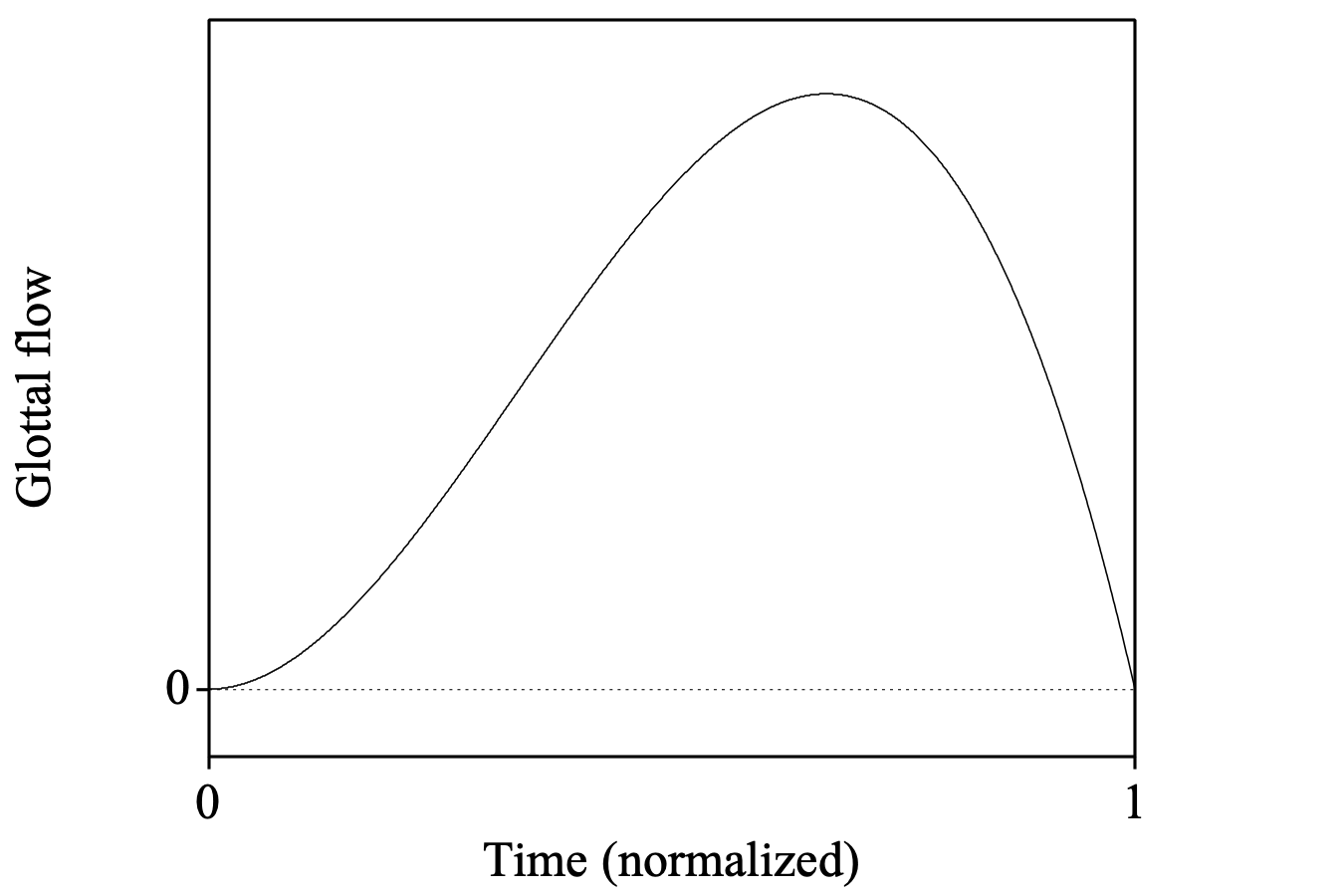If power1 = 3.0 and power2 = 4.0, the glottal flow shape starts somewhat smoother, reflecting the idea that the glottis opens like a zipper: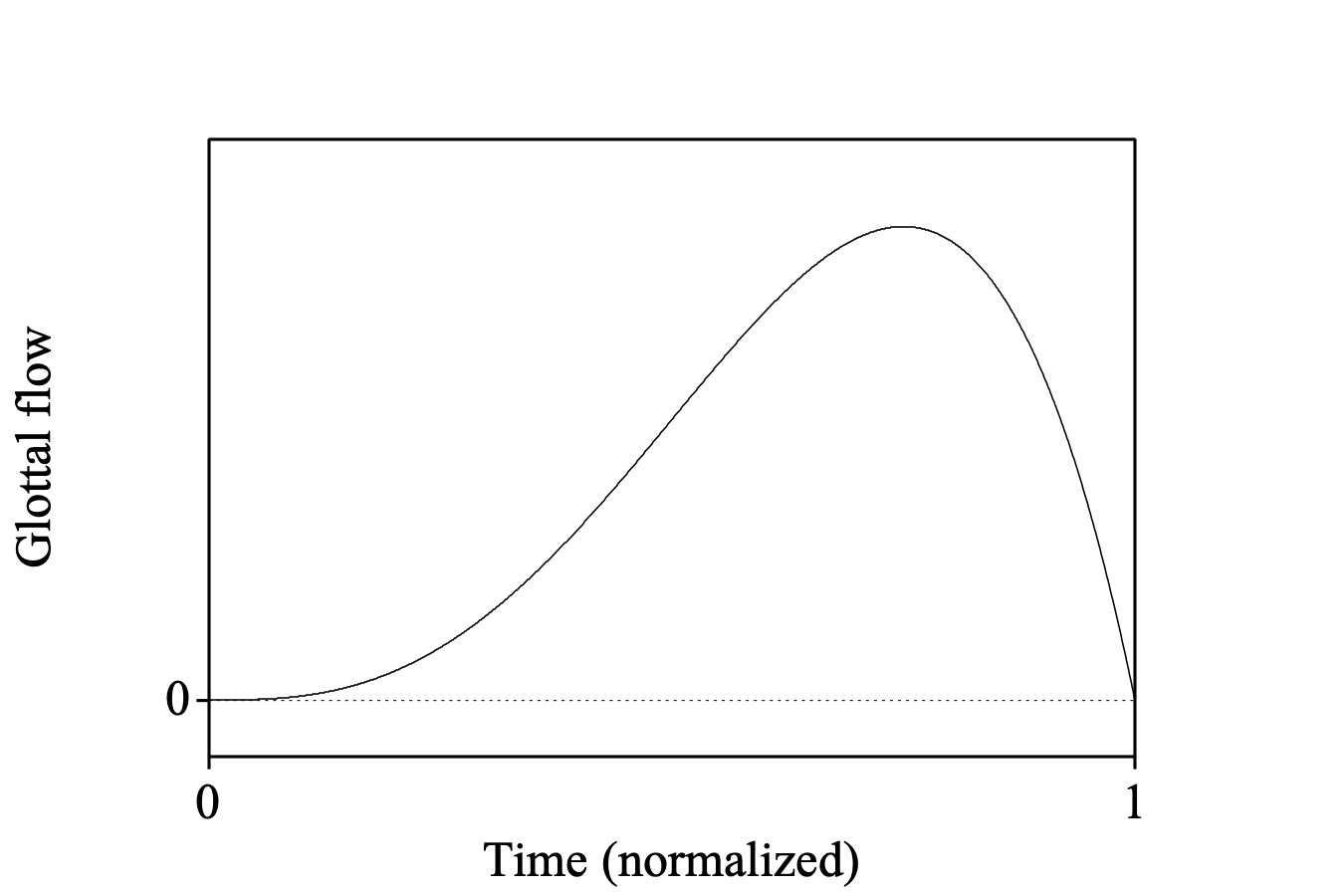For the generation of speech sounds, we do not take the glottal flow itself, but rather its derivative (this takes into account the influence of radiation at the lips). The glottal flow derivative is given by

 dU(x)/dx = power1 x(power1-1) - power2 x(power2-1)

The flow derivative clearly shows the influence of the smoothing mentioned above. The unsmoothed curve, with power1 = 2.0 and power2 = 3.0, looks like: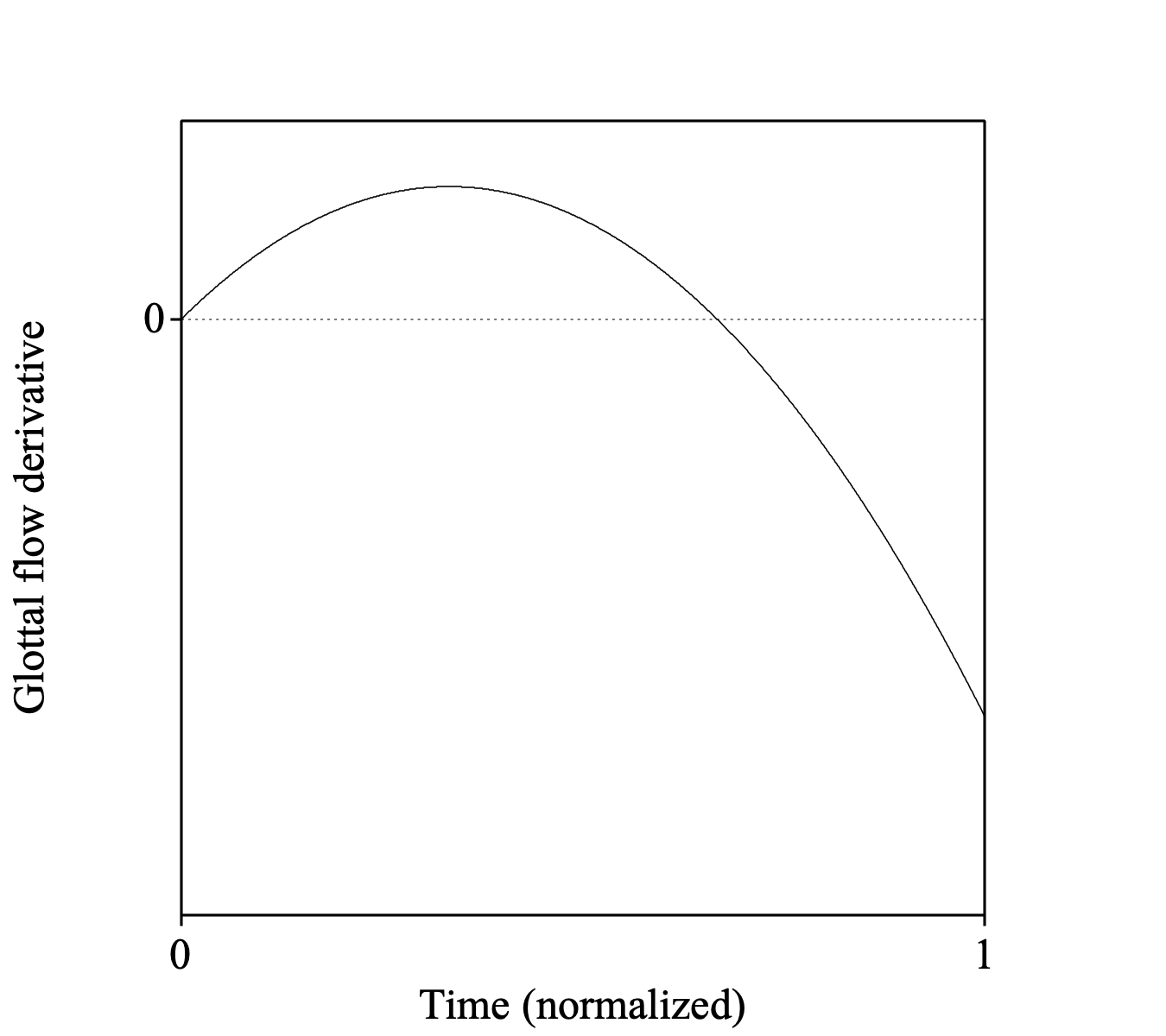Unlike the unsmoothed curve, the smoothed curve, with power1 = 3.0 and power2 = 4.0, starts out horizontally: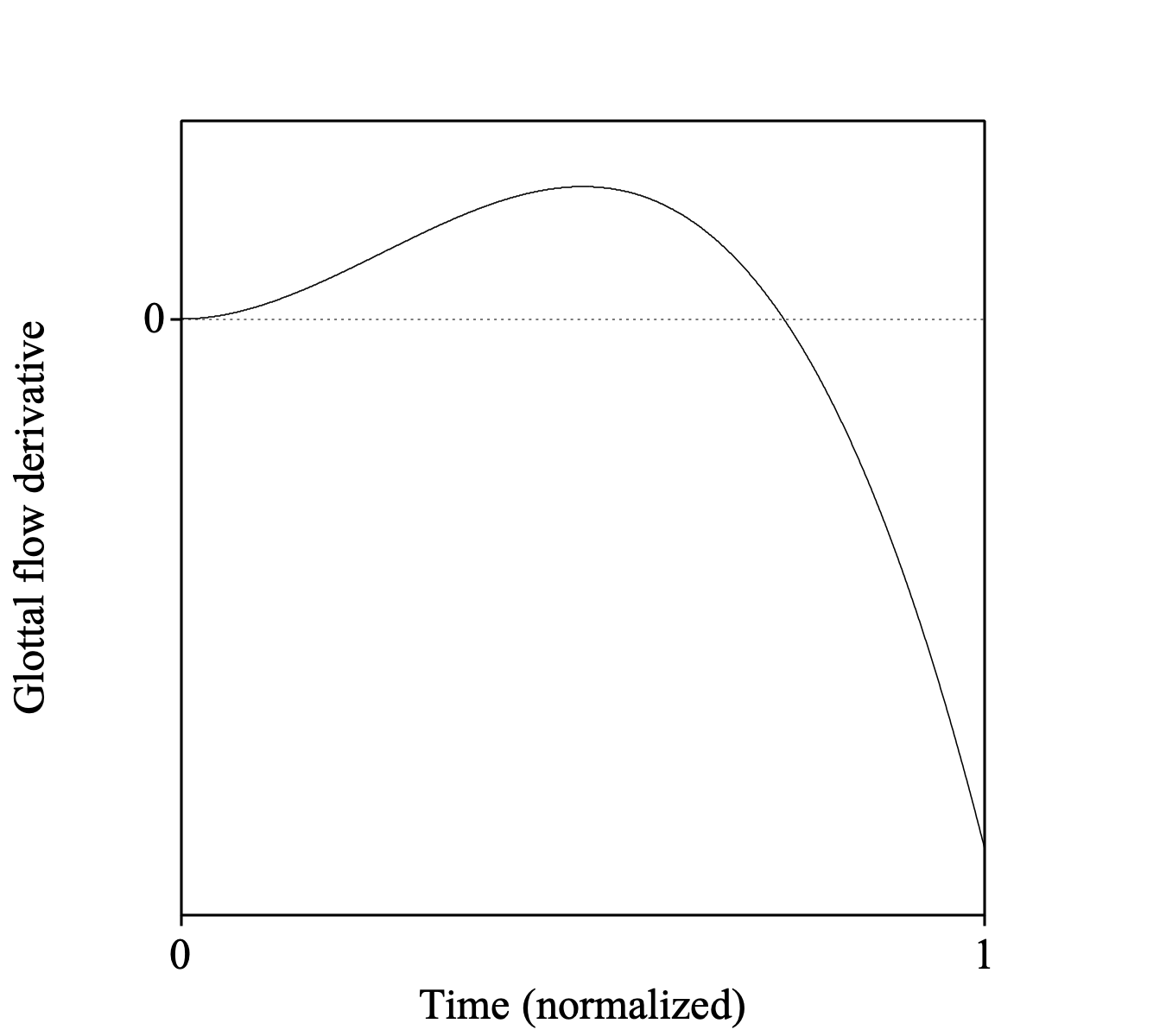Another setting is the open phase. If it is 0.70, the glottis will be open during 70 percent of a period. Suppose that the PointProcess has a pulse at time 0, at time 1, at time 2, and so on. The pulses at times 1 and 2 will then be turned into glottal flows starting at times 0.30 and 1.30:The final setting that influences the shape of the glottal flow is the collision phase. If it is 0.03, for instance, the glottal flow derivative will not go abruptly to 0 at a pulse, but will instead decay by a factor of e (≈ 2.7183) every 3 percent of a period. In order to keep the glottal flow curve smooth (and the derivative continuous), the basic shape discussed above has to be shifted slightly to the right and truncated at the time of the pulse, to be replaced there with the exponential decay curve; this also makes sure that the average of the derivative stays zero, as it was above (i.e. the area under the positive part of the curve equals the area above the negative part). This is what the curves look like if power1 = 3.0, power2 = 4.0, openPhase = 0.70 and collisionPhase = 0.03: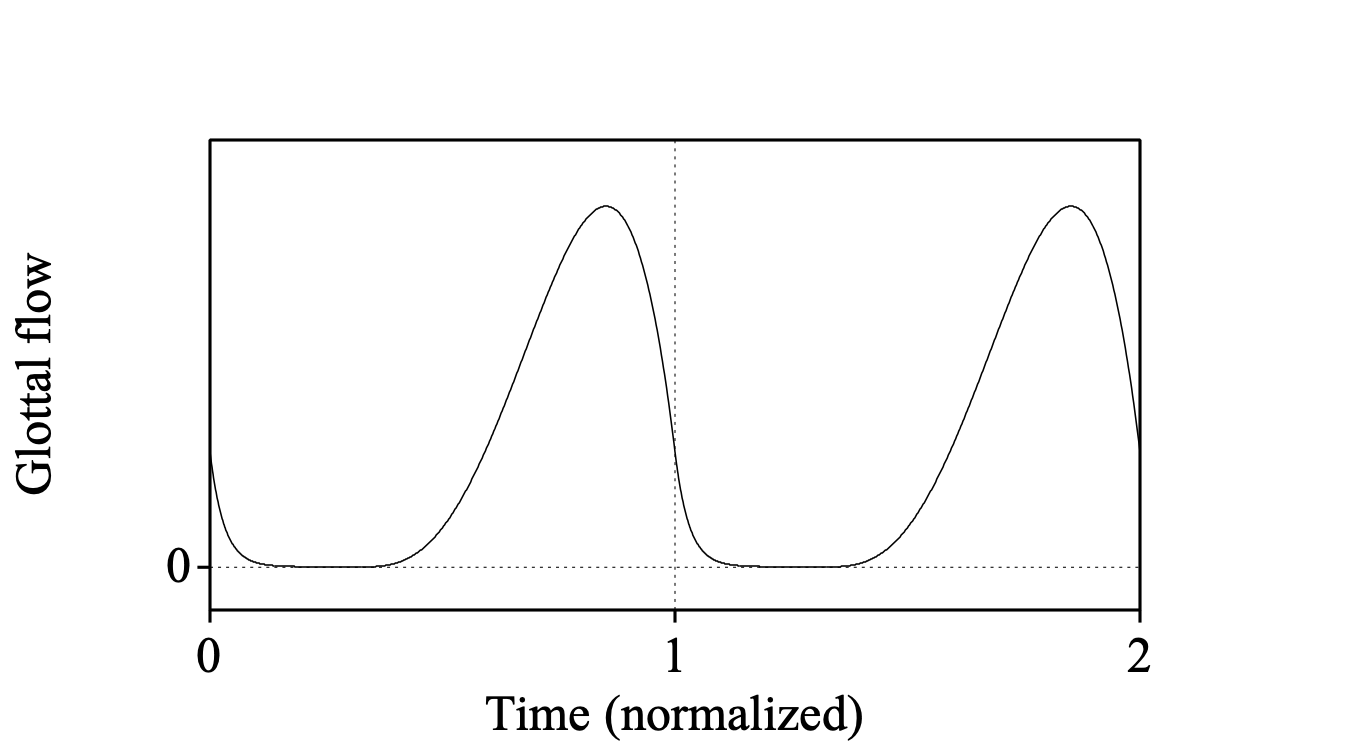These curves have moved 2.646 percent of a period to the right. At time 1, the glottal flow curve turns from a convex polynomial into a concave exponential, and the derivative still has its minimum there.

### Settings

Sampling frequency (Hz)
the sampling frequency of the resulting Sound object, e.g. 44100 hertz.